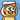# 有多少种硬币组合，更优解法

November 02, 2018

# 问题和复制粘贴的分析

1. 10 = 10
2. 50 = 50
3. 100 = 100
4. 10 + 50 = 60
5. 10 + 100 = 110
6. 50 + 50 = 100
7. 50 + 100 = 150
8. 10 + 50 + 50 = 110
9. 10 + 50 + 100 = 160
10. 50 + 50 + 100 = 200
11. 10 + 50 + 50 + 100 = 210

# 接下来是正经分析

## 第一步：将所有硬币组成一个数组

```/** * Count number of * @param {Array<Number>} coins array contains coins with different values * @param {Array<Number>} counts array contains corresponding counts of different coins * @returns {Number} The count of distinct sums */ function countDistinctSum(coins, counts) { if (coins.length !== counts.length) { return -1; } const results = {}; const coinList = []; for (let i = 0; i < coins.length; i++) { for (let j = 0; j < counts[i]; j++) { coinList.push(coins[i]); } } // where the beauty of algorithm shows return Object.keys(results).length - 1; // Remove the empty 0 coin element }```

## 第二步：了解所有的组合是如何计算得到的

`A + B`

A + B

`A`

A + B

A

`B`

### 三枚硬币：A、B、C

`A + B + C`

`A + C`

`B + C`

A + B + C

A + C

B + C

`A + B`

`A`

`B`

A + B + C

A + C

B + C

A + B

A

B

`C`

• 1 枚硬币时，组合数量是`1`
• 2 枚硬币时，组合数量是`1 * 2 + 1 = 3`
• 3 枚硬币时，组合数量是`3 * 2 + 1 = 7`
• 4 枚硬币时，组合数量是`7 * 2 + 1 = 15`

## 第三步：只储存非重复的值

• `10 + 50 = 60`
• `50 + 50 = 100`
• `60 + 50 = 110`

# 大功告成

```/* * Count number of * @param {Array<Number>} coins array contains coins with different values * @param {Array<Number>} counts array contains corresponding counts of different coins * @returns {Number} The count of distinct sums */ function countDistinctSum(coins, counts) { if (coins.length !== counts.length) { return -1; } const coinList = []; for (let i = 0; i < coins.length; i++) { for (let j = 0; j < counts[i]; j++) { coinList.push(coins[i]); } } // Create a new set const results = new Set(); for (let i = 0; i < coinList.length; i++) { // tempSum is used to store the temporary sum of every element in the set and new coin value const tempSum = []; for (let it = results.values(), val = null; (val = it.next().value); ) { tempSum.push(val + coinList[i]); } // add every sum in tempSum to the set and the set will do automatic duplication removal. tempSum.forEach(n => { results.add(n); }); // add the new coin value into the set results.add(coinList[i]); } return results.size; }```

```const a = [10, 50, 100]; const b = [1, 2, 1]; console.log("Result:", countDistinctSum(a, b)); // Result: 9```Written by Yi Zhiyue
A Software Engineer · 山不在高，有仙则灵